Consider a plane surface of area ΔS in a uniform electric field E in the space. Draw a positive normal to the surface and θ be the angle between electric field E and the normal to the plane.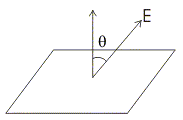Electric flux of the electric field through the chosen surface is then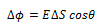Corresponding to area ΔS we can define area vector ΔS of magnitude ΔS along the positive normal. With this definition one can write electric flux under the direction of area vector is always along normal to the surface being chosen.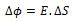Thus electric flux is a measure of lines of force passing through the surface held in the electric field.

#### Special Cases:

• If E is perpendicular to the surface i.e., parallel to the area vector then θ = 0.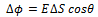• If θ = π i.e., electric field vector is in the direction opposite to area vector then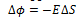• If electric field and area vector are perpendicular to each other then θ = π/2 and ∆ϕ = 0.
• Flux is a scalar quantity and it can be added using rules of scalar addition.
• The unit of electric flux is NC-1 m2.